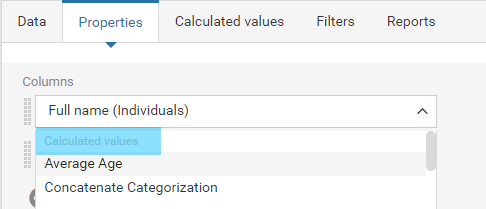Most users use this field to calculate arithmetic functions such as count, sum, minimum value, maximum value, average.

First, select what to calculate in the drop-down list and choose which function to apply. Various functions are available, depending on the type of field selected (number, text, values from a selection list, etc.)

Depending on the value you choose, you can calculate in 9 different ways:

• Average: Only available for numeric fields. Calculates the average number of all the entities for that field.

• Concatenate: Links together a chain of values in a single row.

• Count: Adds the different entities related to the selected field. Each occurrence is counted once.

• Cumulative count: Adds the different entities related to the selected field (including the sum of values in rows above).

• Cumulative sum: Only available for numeric fields. Adds the numbers from all the entities found in the selected field (including the sum of values in rows above).

• Maximum: Only available for numeric fields and dates. Identifies the highest number found or latest date for that field.

• Median: Only available for numeric fields. Identifies the middle number of a given sequence.

• Minimum: Only available for numeric fields and dates. Identifies the smallest number found or earliest date for that field.

• Sum: Only available for numeric fields. Calculates the sum of the numbers from all the entities found in that field.

The name of the calculated value has to be selected from the drop-down of columns/categories in order to display in the widget. The name will always appear at the beginning of the list.